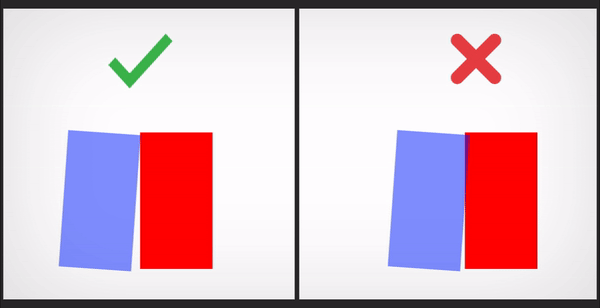# How to avoid overlapping of GameObject without rigidbody?

I was looking for a way to make my gameobject not overlap to the another gameobject but all the solutions are talking about Rigidbody…
_
when i want to do it in script only, is it possible ? I have a cube with this scale (3,1,1) I make him rotate around itself but i got the overlap problem because his x scale is 3

Is there anyway to make him move away automatically to avoid the red gameobject?
_If this is a special case where your objects are always in this sort of relative location and just want to avoid overlapping with one other specific object then you could code a reasonably simple solution yourself. However any variation in how you want this to work will massively increase the code complexity, and it would then be better to use rigidbodies and joints etc.

The simple solution for this setup could be to find the bounding box of your rotated object in worldspace, and offset your position based on the diff between the current world bounding box and the starting position world bounding box, here’s some working, but improvable code!

``````using UnityEngine;
using System.Collections;
using System.Collections.Generic;
using System.Linq;

public class HomeGrownPhysics : MonoBehaviour {

Transform t;
List<Vector3> localCorners;
float xLock;
float xPos;

// Use this for initialization
void Start () {
CalculateLocalValues();
xLock = CalculateWorldBounds(t).extents.x;
xPos = t.position.x;
}

// Update is called once per frame
void Update () {
t.RotateAround(t.position, Vector3.forward, Time.deltaTime * 10.0f);

Bounds b = CalculateWorldBounds(t);
t.position = new Vector3(xPos + xLock - CalculateWorldBounds(t).extents.x, t.position.y, t.position.z);
}

private void CalculateLocalValues()
{
t = transform;
Bounds bounds = this.GetComponent<MeshFilter>().mesh.bounds;

Vector3 centre = bounds.center;
Vector3 M__ = new Vector3(bounds.extents.x, 0, 0);
Vector3 _M_ = new Vector3(0, bounds.extents.y, 0);
Vector3 __M = new Vector3(0, 0, bounds.extents.z);

Debug.Log("Centre " + centre);

localCorners = new List<Vector3> {
centre - M__ - _M_ - __M
,centre - M__ - _M_ + __M
,centre - M__ + _M_ - __M
,centre - M__ + _M_ + __M
,centre + M__ - _M_ - __M
,centre + M__ - _M_ + __M
,centre + M__ + _M_ - __M
,centre + M__ + _M_ + __M
};
}

private Bounds CalculateWorldBounds(Transform transform)
{
Matrix4x4 transformation = transform.localToWorldMatrix;

List<Vector3> corners = localCorners.Select(x => transformation.MultiplyPoint3x4(x)).ToList();
Vector3 min = corners.Aggregate((a, b) => Vector3.Min(a, b));
Vector3 max = corners.Aggregate((a, b) => Vector3.Max(a, b));

Vector3 diff = max - min;
return new Bounds(min + (diff/2.0f), diff);
}

}
``````